Like   Tweet   Pin   +1   in/* styles */ Tips & resources on wellbeing & performance   ||   Issue #40, February 2022
 table div table+table+table div table{width:100%;padding:0}table div table+table+table div table img{width:96.23%;padding:0;float:none}table div table+table+table div table td{width:100%;padding:0 1.88% 18px}/* styles */In this issue there's a post on what back to 'normal' means to me, a Tai Chi tip and a Lao Tzu quote. There are also updates on my Sports Chi and Chair Chi Plus programs.

And I've included a short YouTube clip on Ma Bu (Tai Chi training).

Regards
Chris Bennett
Chris Chi

 table div table+table+table+table+table div table{width:100%;padding:0}table div table+table+table+table+table div table img{width:96.23%;padding:0;float:none}table div table+table+table+table+table div table td{width:100%;padding:0 1.88% 18px}/* styles */## Back to 'Normal'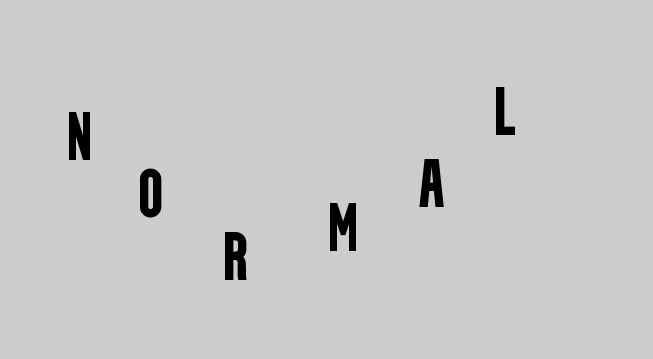Slowly things are getting back to normal, or should I say Covid 'normal'. I'm starting to get bookings from aged care centres again for my Chair Chi sessions for residents.

I'm also looking forward to when I'll be able to run face to face workshops for my Sports Chi program, designed for coaches and players to improve their performances in training and games.

Hopefully, it will be sooner than later.

 table div table+table+table+table+table+table+table+table div table{width:100%;padding:0}table div table+table+table+table+table+table+table+table div table img{width:96.23%;padding:0;float:none}table div table+table+table+table+table+table+table+table div table td{width:100%;padding:0 1.88% 18px}/* styles */## Sports Chi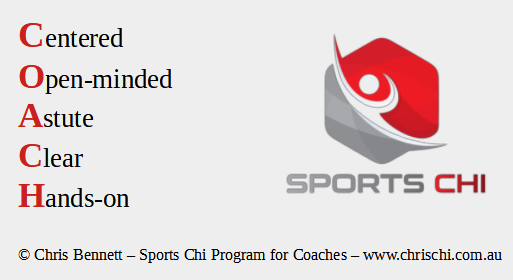Getting there. I'm working on my online Sports Chi program for coaches and just created the C.O.A.C.H acronym. The online course comes from my several years of experience as a Tai Chi teacher and basketball coach.

 table div table+table+table+table+table+table+table+table+table+table+table div table{width:100%;padding:0}table div table+table+table+table+table+table+table+table+table+table+table div table img{width:96.23%;padding:0;float:none}table div table+table+table+table+table+table+table+table+table+table+table div table td{width:100%;padding:0 1.88% 18px}/* styles */## Tai Chi Tip

 /* styles */ When practicing your horse stance (high, medium or low) avoid collapsing your knees inwardly and rolling your feet outwardly.
 table div table+table+table+table+table+table+table+table+table+table+table+table+table+table div table{width:100%;padding:0}table div table+table+table+table+table+table+table+table+table+table+table+table+table+table div table img{width:96.23%;padding:0;float:none}table div table+table+table+table+table+table+table+table+table+table+table+table+table+table div table td{width:100%;padding:0 1.88% 18px}/* styles */## Chair Chi Plus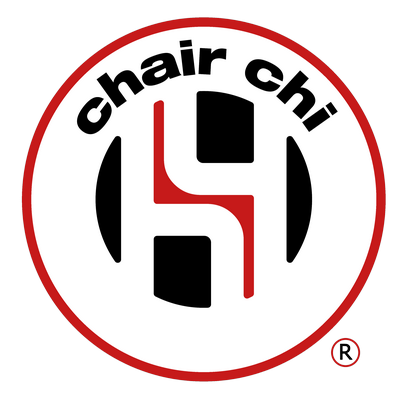I'm revamping Chair Chi Plus. This is part of our overall Chair Chi (seated Tai Chi) , three level, training program for aged care staff.

Over past few years I've been running public workshops, but now I offer the workshops onsite only.

 table div table+table+table+table+table+table+table+table+table+table+table+table+table+table+table+table+table div table{width:100%;padding:0}table div table+table+table+table+table+table+table+table+table+table+table+table+table+table+table+table+table div table img{width:96.23%;padding:0;float:none}table div table+table+table+table+table+table+table+table+table+table+table+table+table+table+table+table+table div table td{width:100%;padding:0 1.88% 18px}/* styles */## Mind and Body Challenge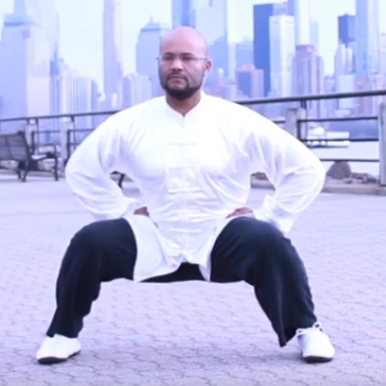The Ma Bu (horse stance) demonstrated by a member of the Hong Kong Tai Chi Qigong organisation is very demanding. The 2.22m clip is best viewed without the sound.

I practice this as part of my Tai Chi training and it's an effective way of learning how to calm your mind under intense physical pressure.

Please note, it should only be learned from a capable instructor in order to avoid injury.

 table div table+table+table+table+table+table+table+table+table+table+table+table+table+table+table+table+table+table+table+table div table{width:100%;padding:0}table div table+table+table+table+table+table+table+table+table+table+table+table+table+table+table+table+table+table+table+table div table img{width:96.23%;padding:0;float:none}table div table+table+table+table+table+table+table+table+table+table+table+table+table+table+table+table+table+table+table+table div table td{width:100%;padding:0 1.88% 18px}/* styles */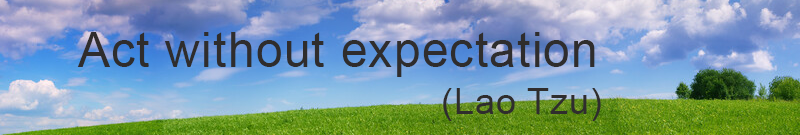table div table+table+table+table+table+table+table+table+table+table+table+table+table+table+table+table+table+table+table+table+table+table div table{width:100%;padding:0}table div table+table+table+table+table+table+table+table+table+table+table+table+table+table+table+table+table+table+table+table+table+table div table img{width:96.23%;padding:0;float:none}table div table+table+table+table+table+table+table+table+table+table+table+table+table+table+table+table+table+table+table+table+table+table div table td{width:100%;padding:0 1.88% 18px}/* styles *//* styles */
 table div table+table+table+table+table+table+table+table+table+table+table+table+table+table+table+table+table+table+table+table+table+table+table+table div table{width:100%;padding:0}table div table+table+table+table+table+table+table+table+table+table+table+table+table+table+table+table+table+table+table+table+table+table+table+table div table img{width:96.23%;padding:0;float:none}table div table+table+table+table+table+table+table+table+table+table+table+table+table+table+table+table+table+table+table+table+table+table+table+table div table td{width:100%;padding:0 1.88% 18px}/* styles *//* styles */